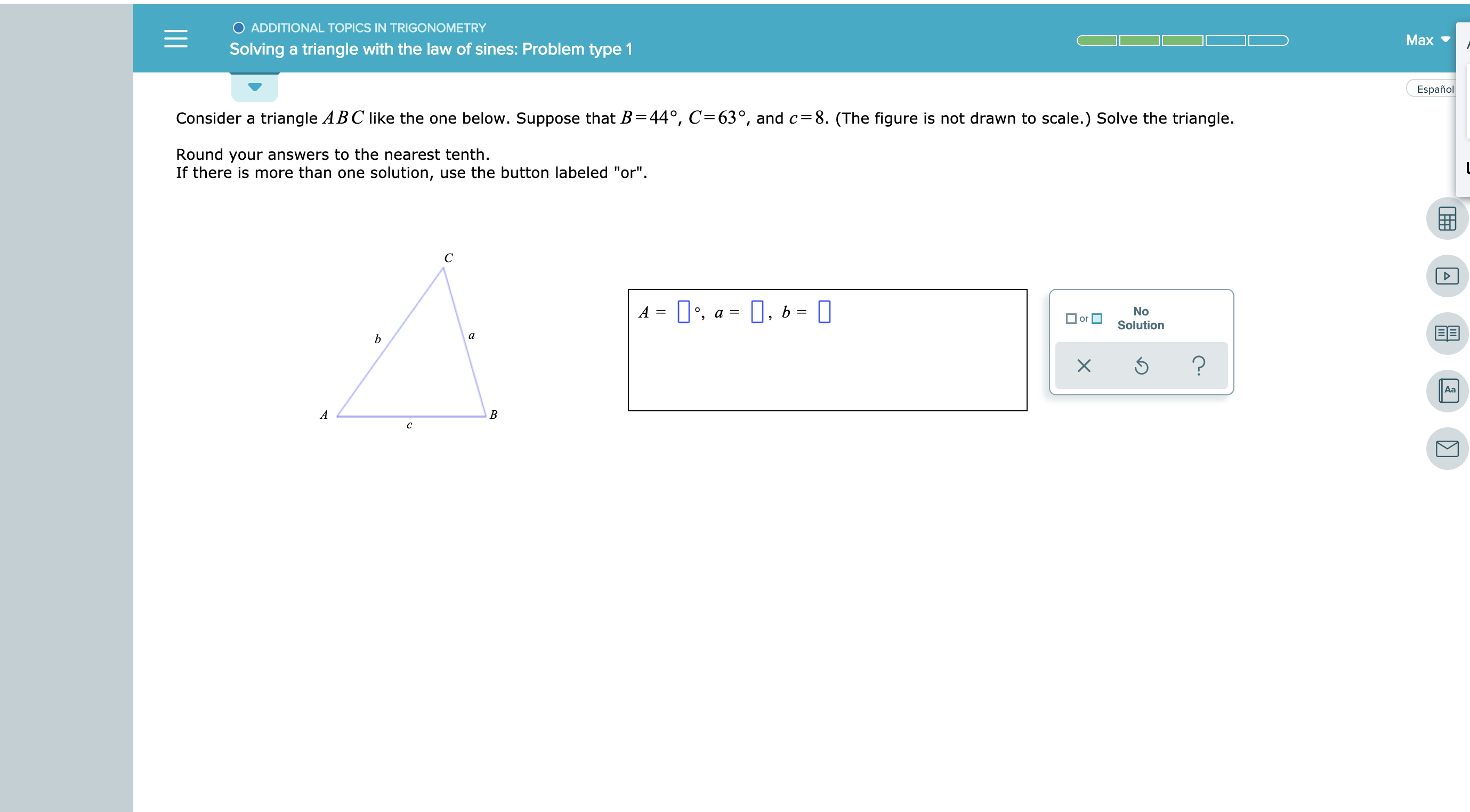# O ADDITIONAL TOPICS IN TRIGONOMETRYMaxSolving a triangle with the law of sines: Problem type 1EspañolConsider a triangle ABC like the one below. Suppose that B =44°, C=63°, and c=8. (The figure is not drawn to scale.) Solve the triangle.Round your answers to the nearest tenth.If there is more than one solution, use the button labeled "or"С, a, bANoSolutionor?XAaABC

Question
1 views

see attachedhelp_outlineImage TranscriptioncloseO ADDITIONAL TOPICS IN TRIGONOMETRY Max Solving a triangle with the law of sines: Problem type 1 Español Consider a triangle ABC like the one below. Suppose that B =44°, C=63°, and c=8. (The figure is not drawn to scale.) Solve the triangle. Round your answers to the nearest tenth. If there is more than one solution, use the button labeled "or" С , a , b A No Solution or ? X Aa A B C fullscreen
check_circle

Step 1

The angle A is computed by the formula, the sum of ...

### Want to see the full answer?

See Solution

#### Want to see this answer and more?

Solutions are written by subject experts who are available 24/7. Questions are typically answered within 1 hour.*

See Solution
*Response times may vary by subject and question.
Tagged in

### Other